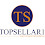## 27/11/2017

### Atomic Structure

In this theory, Neil Bohr’s atomic structure, definitions of matter, molecules, atoms, nucleus, atomic weight, extra nucleus, electrons in orbit, atomic number and energy of an electron are explained.

# Atomic structure

• The scientist Neil Bohr explains the atomic structure.
• According to Neil an atom consists of positive charged nucleus and negative charged electrons revolve in circular orbit.
• The electrons can revolve around the nucleus in certain orbits.
• Each orbit consists of certain energy level.
• As the distance between nucleus and orbit increases, energy of electrons increases.
• If the energy is given to an electron, the electron lifts to higher energy level. This is called as excitation.

## Matter

• The matter is defined as anything which occupies space and weight such as wood, copper, water, air etc.

## Molecule

• The matter is made up of very small particles known as molecules.

## Atoms

• It is smallest into which matter can be divided without release of charged particles. It is smallest unit of matter.

## Nucleus

• The nucleus of an atom is mainly consists of two particles protons and neutrons.
• The proton has positive charge whereas the neutron has no charge. The mass of neutron is same as that of proton.
• Therefore the nucleus has positive charge.

## Atomic weight

• It is sum of weight of protons and weight of neutrons.
• Atomic weight = weight of protons + weight of neutrons
• A = Z + N
• According to electron theory, the atoms of all elements composed mainly two parts namely nucleus at the center and electrons surrounding it.
• The nuclear symbol consists of three parts, symbol of element, atomic number and atomic mass.
• Let us try to understand it.

26Al13 = A Al Z = Number of protons + Number of neutrons Al Number of protons

Mass of aluminum (A) is 26

Atomic number of aluminum (Z) is 13

Therefore the number of neutron in the aluminum = A – Z = 26 – 13

= 13

## Extra nucleus (Electrons)

• It is outer part of an atom which contains electrons only.
• The charge of an electron is equal and opposite to that of charge of proton. The number of electron is equal to number of proton.
• Therefore we can say that an atom is neutral.
• Charge of an electron = 1.602 × 10 – 19 Coulomb
• Mass of an electron = 9.0 × 10 – 19 Kg
• Radius of an electron = 1.9 × 10 – 15 Meter
• The ratio of charge of an electron to the mass of an electron is 1.77 × 1011 Coulomb per kg.
• It means that mass of an electron is very small as compared to its charge. The electron is greatly affected by electric or magnetic field.

## Electrons in orbit

• The electrons of an atom around nucleus revolve in different orbits.
• The number of electrons in any orbit is equal to 2n2.

Where n is orbit number

Number of electrons in first orbit = 2(1)2 = 2

Second orbit = 2(2)2 = 8

Third orbit = 2(3)2 = 18

• The number of electron in the last orbit should not greater than 8
• Any orbit should not contain more than 18 electrons.
• The atomic number of silicon is 14. It means that 2 electrons lies in first orbit, 8 electrons in second orbit and remaining 4 electrons in the third or last orbit.

## Atomic number

• It is defined as the number of proton is equal to number of electrons.

Atomic number = Number of proton

= Number of electrons

• The atomic number of sodium is eleven (11), it means that it has 11 number of protons and 11 number of electrons.
• It has 2 electronics in first orbit, 8 electrons in the second orbit and only one electron in the last or third orbit. List of number is given here.

https://education.jlab.org/itselemental/index_num.html

## Energy of an electron

• Each electron consists of kinetic energy due to its motion and potential energy due to charge of electron.
• As the distance between nucleus and electron increases, the energy of an electron also increases. Therefore
• Energy of 1st orbit electrons < Energy of 2nd orbit electrons
• Energy of 2nd orbit electrons < Energy of 3rd orbit electrons
• Energy of 3rd orbit electrons < Energy of 4th orbit electrons
• It means that electrons of first orbit consists lowest energy whereas the electrons of last orbit have highest energy.
• The electrons of last orbit play important role to determine electrical, chemical and physical properties of a material.

You may also like :

1.Now day, everything is going to find a new but well settled and successful stream for their career. When I came to this blog, I really impressed by all the knowledge points mentioned here. Thank you for this assistance.dell server ราคา

2.We need all the more such articles that we can read with such excitement.
southend electrician

3.Fortunately, if you're just looking to get rid of some old or broken electronic devices, you don't need to go to those lengths.electronic components uk

4.Awesome work! That is quite appreciated. I hope you’ll get more success.
instrument transformers

5.Thanks for a wonderful share. Your article has proved your hard work and experience you have got in this field. Brilliant .i love it reading. Learn more. Electricity